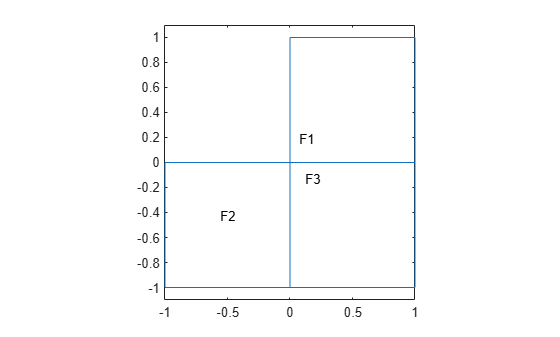# findElectromagneticBC

Find electromagnetic boundary conditions assigned to geometric region

## Syntax

``emBC = findElectromagneticBC(emagmodel.BoundaryConditions,RegionType,RegionID)``

## Description

example

````emBC = findElectromagneticBC(emagmodel.BoundaryConditions,RegionType,RegionID)` returns the boundary conditions assigned to the specified region of the specified model.```

## Examples

collapse all

Create an electromagnetic model for magnetostatic analysis.

`emagmodel = createpde("electromagnetic","magnetostatic");`

Include an L-shaped membrane geometry in the model and plot it with the face labels.

```geometryFromEdges(emagmodel,@lshapeg); pdegplot(emagmodel,"FaceLabels","on") ylim([-1.1 1.1]) axis equal```Assign magnetic potential values to edges 1 and 2.

```electromagneticBC(emagmodel,"Edge",1,"MagneticPotential",1); electromagneticBC(emagmodel,"Edge",2,"MagneticPotential",0);```

Check the boundary condition specifications for edges 1 and 2.

```emBC = findElectromagneticBC(emagmodel.BoundaryConditions,"Edge",1:2); emBC(1)```
```ans = ElectromagneticBCAssignment with properties: MagneticPotential: 1 RegionID: 1 RegionType: 'Edge' Vectorized: 'off' Voltage: [] ```
`emBC(2)`
```ans = ElectromagneticBCAssignment with properties: MagneticPotential: 0 RegionID: 2 RegionType: 'Edge' Vectorized: 'off' Voltage: [] ```

Create an electromagnetic model for electrostatic analysis.

`emagmodel = createpde("electromagnetic","electrostatic");`

Import and plot a geometry representing a plate with a hole.

```gm = importGeometry(emagmodel,"PlateHoleSolid.stl"); pdegplot(gm,"FaceLabels","on","FaceAlpha",0.3)```Apply the voltage boundary conditions on the side faces and the face bordering the hole.

```electromagneticBC(emagmodel,"Voltage",0,"Face",3:6); electromagneticBC(emagmodel,"Voltage",1000,"Face",7);```

Check the boundary condition specifications for faces 4, 5, and 7.

```emBC = findElectromagneticBC(emagmodel.BoundaryConditions, ... "Face",[4 5 7]); emBC(1)```
```ans = ElectromagneticBCAssignment with properties: MagneticPotential: [] RegionID: [3 4 5 6] RegionType: 'Face' Vectorized: 'off' Voltage: 0 ```
`emBC(2)`
```ans = ElectromagneticBCAssignment with properties: MagneticPotential: [] RegionID: [3 4 5 6] RegionType: 'Face' Vectorized: 'off' Voltage: 0 ```
`emBC(3)`
```ans = ElectromagneticBCAssignment with properties: MagneticPotential: [] RegionID: 7 RegionType: 'Face' Vectorized: 'off' Voltage: 1000 ```

## Input Arguments

collapse all

Boundary conditions of an electromagnetic model, specified as the `BoundaryConditions` property of the model.

Example: `findElectromagneticBC(emagmodel.BoundaryConditions,"Edge",1)`

Geometric region type, specified as `"Edge"` for a 2-D model or `"Face"` for a 3-D model.

Data Types: `char` | `string`

Region ID, specified as a vector of positive integers. Find the edge or face IDs by using `pdegplot` with the `"EdgeLabels"` or `"FaceLabels"` name-value argument set to `"on"`.

Data Types: `double`

## Output Arguments

collapse all

Electromagnetic boundary condition assignment, returned as an `ElectromagneticBCAssignment` object. For more information, see ElectromagneticBCAssignment Properties.

## Version History

Introduced in R2021a